Composite Fermat number implies Poulet number

Statement

Supposeis a nonnegative integer. Let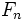be theFermat number:.

Then: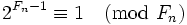.

In particular, ifis composite, thenis a Poulet number.

Proof

Given: A nonnegative integer,.

To prove:.

Proof: For any nonnegative integer,. Thus,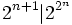.

Now, for a Fermat number, the order ofmodulois precisely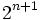. From the above, we conclude that the order ofmodulodivides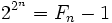, so.